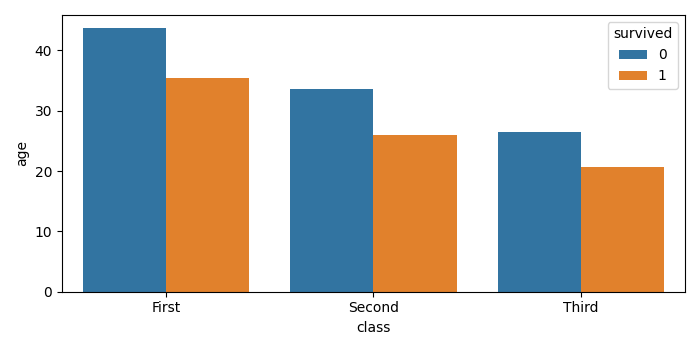# How to turn off error bars in Seaborn Bar Plot using Matplotlib?

To turn off error bars in a Seaborn bar plot, we can take the following steps−

• Load an example dataset from the online repository (requires Internet).
• Show the point estimates and confidence intervals with bars.
• To display the figure, use show() method.

## Example

import seaborn as sns
import matplotlib.pyplot as plt
plt.rcParams["figure.figsize"] = [7.00, 3.50]
plt.rcParams["figure.autolayout"] = True
plt.show()